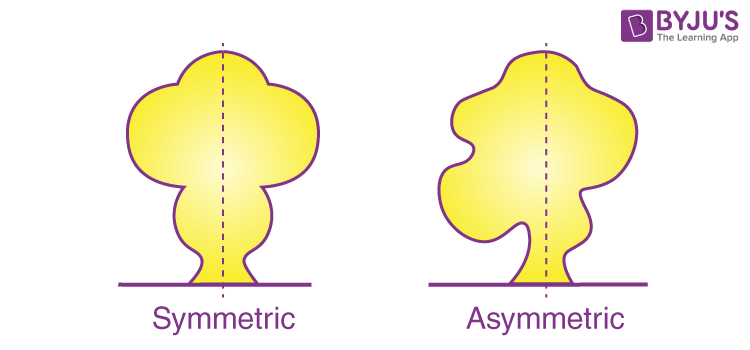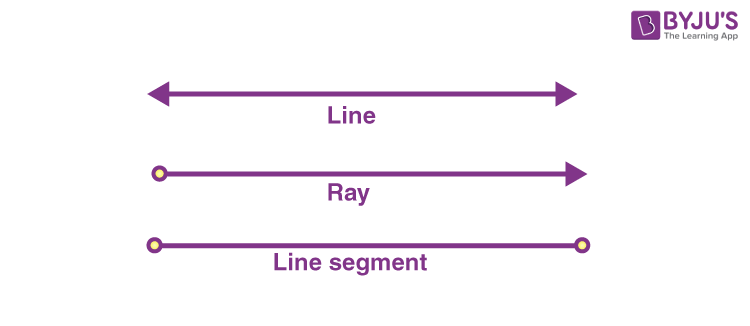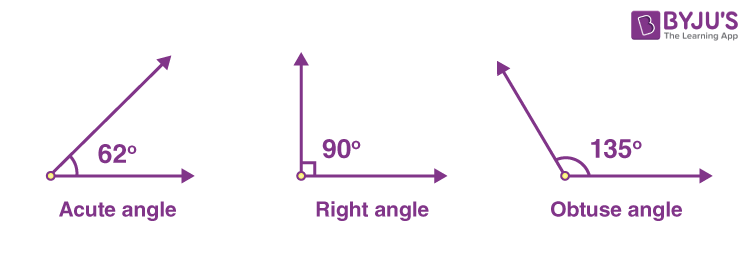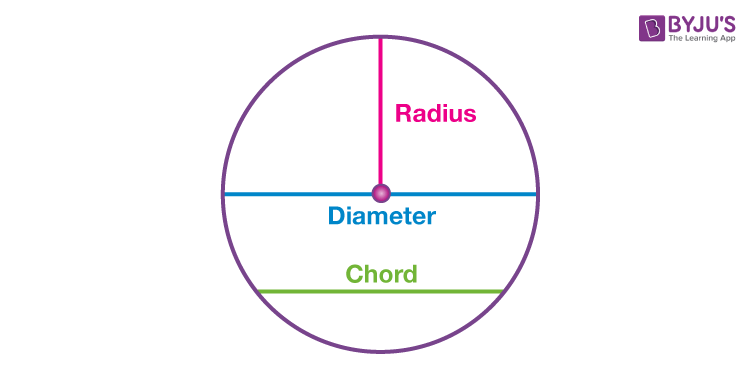# Geometry

In our previous class, we‌ ‌have‌ ‌learned‌ ‌about essential elements of Geometry, such as points, lines, and some geometrical shapes ‌.‌ ‌

In this chapter, we will be learning about the line of symmetry, line segment, ray, angles and circular figures.

We see many objects around us, such as pencils, erasers, boxes, water bottles, windows, doors, lunch boxes, wall clocks, blackboards, etc. All these objects have different sizes and shapes. While a few are long, round and four-sided squares, others are rectangular, and a lot more.

To know the various properties of these objects, let us understand some basic geometrical facts.

## Symmetry and Asymmetrical Objects

Some objects have plane surfaces, some have a round surface, whereas others have both types of plane surfaces.

• A lunch box, a postcard, a tabletop and a top surface of a book are a few objects with plane surfaces.
• Table lamps, bowls, vases, and flower pots are objects with round or curved surfaces.
• When a straight line divides a figure into exactly two equal parts, such figures are called symmetrical, and the line dividing this figure is called the line of symmetry.
• Figures, which are not divided into two equal parts by a straight line, are asymmetrical.### Line, Ray and Line SegmentThe least distance between two points is called a line segment.

If the line segment is extended indefinitely on both sides, it is called a line.

If the line segment is extended from its endpoint to an infinite length in the same direction, it is called a ray.

Straight lines, curved lines, parallel lines and perpendicular lines are different types of lines.

### Angle and Types of Angle

When two rays are connected at their endpoints, an angle is formed.

The symbol of angle is ‘<.’ Angles are measured in degrees (°), using a protractor.

Types of AnglesAcute angle

An angle whose measure is less than 90°, is called an acute angle.

Right angle

An angle whose measure is 90°, is called a right angle.

Obtuse angle

An angle whose measure is more than 90° and less than 180°, is called an obtuse angle.

### CirclesA curve drawn from a fixed point, keeping the distance constant, is called a circle.

• The diameter is the longest chord of the circle.
• The length of the diameter of a circle is twice the length of the radius.
• The fixed point around which the circle is drawn is called the centre of the circle.
• The segment joining any two points on the circle’s circumference is called a chord.
• The measurement of the boundary of the circle is called the circumference or perimeter of the circle.
• All the points on the circle are at the same distance from the centre. This distance is called the radius of the circle.

Stay tuned to BYJU’S for more information on NIOS, syllabus, notes, along with its important questions and solutions